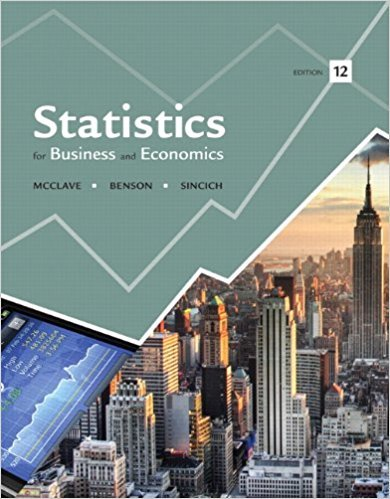×
×

# Solved: Do social robots walk or roll? Refer to theISBN: 9780321826237 51

## Solution for problem 37E Chapter 3

Statistics for Business and Economics | 12th Edition

• Textbook Solutions
• 2901 Step-by-step solutions solved by professors and subject experts
• Get 24/7 help from StudySoup virtual teaching assistantsStatistics for Business and Economics | 12th Edition

4 5 0 344 Reviews
31
3
Problem 37E

Do social robots walk or roll? Refer to the International Conference on Social Robotics (Vol. 6414, 2010) study of the trend in the design of social robots, Exercise 2.3 (p. 48). Recall that in a random sample of 106 social (or service) robots designed to entertain, educate, and care for human users, 63 were built with legs only, 20 with wheels only, 8 with both legs and wheels, and 15 with neither legs nor wheels. One of the 106 social robots is randomly selected and the design (e.g., wheels only) is noted.

a. List the sample points for this study.

b. Assign reasonable probabilities to the sample points.

c. What is the probability that the selected robot is designed with wheels?

d. What is the probability that the selected robot is designed with legs?

e. Use the rule of complements to find the probability that the selected robot is designed with either legs or wheels.Step-by-Step Solution:
Step 1 of 3

Solution 37E

Step1 of 6:

From the given problem we have a random sample of 106 social robots designed to entertain, educate, and care for human users.

The number of robots built with legs = 63.

The number of robots built with wheels = 20.

The number of robots built with both legs and wheels = 8.

The number of robots built with neither legs nor wheels = 15.

Here our goal is:

a). We need to list the sample points for this study.

b). We need to assign reasonable probabilities to the sample points.

c). We need to find the probability that the selected robot is designed with wheels.

d). We need to find the probability that the selected robot is designed with legs.

e). We need to find the probability that the selected robot is designed with either legs or wheels.

Step2 of 6:

a).

Let us define the events:

L = Legs only

W = wheels only

B = both legs and wheels

N = neither legs nor wheels.

Then, we have 4 sample points and they are given by:

{L, W, B, N}.

Step3 of 6:

b).

Consider,Hence, probability that the robots built with legs is 0.5943.

Similarly,Hence, probability that the robots built with wheels is 0.1886.

Now,Hence, probability that the robots built with both legs and wheels is...

Step 2 of 3

Step 3 of 3

##### ISBN: 9780321826237

The answer to “Do social robots walk or roll? Refer to the International Conference on Social Robotics (Vol. 6414, 2010) study of the trend in the design of social robots, Exercise 2.3 (p. 48). Recall that in a random sample of 106 social (or service) robots designed to entertain, educate, and care for human users, 63 were built with legs only, 20 with wheels only, 8 with both legs and wheels, and 15 with neither legs nor wheels. One of the 106 social robots is randomly selected and the design (e.g., wheels only) is noted.a. List the sample points for this study.b. Assign reasonable probabilities to the sample points.c. What is the probability that the selected robot is designed with wheels?d. What is the probability that the selected robot is designed with legs?e. Use the rule of complements to find the probability that the selected robot is designed with either legs or wheels.” is broken down into a number of easy to follow steps, and 150 words. Statistics for Business and Economics was written by and is associated to the ISBN: 9780321826237. This full solution covers the following key subjects: wheels, social, Legs, robots, designed. This expansive textbook survival guide covers 15 chapters, and 1630 solutions. The full step-by-step solution to problem: 37E from chapter: 3 was answered by , our top Business solution expert on 07/21/17, 05:42AM. This textbook survival guide was created for the textbook: Statistics for Business and Economics , edition: 12. Since the solution to 37E from 3 chapter was answered, more than 1683 students have viewed the full step-by-step answer.

Unlock Textbook Solution